# Hierarchical Bayesian Aldrich-McKelvey Scaling in R via Stan

The goal of the hbamr package is to enable users to efficiently perform Hierarchical Bayesian Aldrich-McKelvey (HBAM) scaling in R. Aldrich-McKelvey (AM) scaling is a method for estimating the ideological positions of survey respondents and political actors on a common scale using ideological survey data (Aldrich and McKelvey 1977). The hierarchical versions of the AM model included in this package outperform other versions both in terms of yielding meaningful posterior distributions for all respondent positions and in terms of recovering true respondent positions in simulations (Bølstad 2023). The package mainly uses the NUTS algorithm in rstan – a Markov chain Monte Carlo (MCMC) algorithm – to fit these models. However, it also offers a simplified model that can be fit using optimization to analyze large data sets quickly.

This vignette provides an overview of how to use the key functions in the hbamr package. The vignette walks through an applied example, showing how to prepare data, fit models, extract estimates, plot key results, and perform cross-validation.

## Example Data

For illustration, we will use data from the 1980 American National Election Study (ANES). This is the same data set that serves to illustrate the original AM model in the basicspace package. The data set is included in the hbamr package and can be loaded by running data(LC1980).

The data set contains respondents’ placements of themselves and six stimuli on 7-point Liberal-Conservative scales. The stimuli in question are: The Democratic and Republican parties, Democratic presidential candidate Jimmy Carter, Republican candidate Ronald Reagan, independent candidate (and former Republican) John B. Anderson, and Ted Kennedy (who challenged the incumbent Carter, but failed to win the Democratic nomination).

We load the data and re-code missing values as follows:

library("hbamr")
data(LC1980)
LC1980[LC1980 == 0 | LC1980 == 8 | LC1980 == 9] <- NA
self <- LC1980[, 1]
stimuli <- LC1980[, -1]
head(stimuli) 
##    Carter Reagan Kennedy Anderson Republicans Democrats
## 1       2      6       1        7           5         5
## 8       4      6       4        7           6         4
## 9       3      6       3        3           6         2
## 10      6      4       3        3           5         4
## 11      7      2       5        5           7         5
## 13      6      6       2        5           7         4

## Preparing the Data

The function prep_data() serves to prepare the data. This function can be run ahead of fitting the models, or it can be run implicitly as part of a single function call to fit the models (as shown below). The function takes a vector of $$N$$ ideological self-placements and an $$N \times J$$ matrix of stimulus placements. It applies a set of inclusion criteria, performs any necessary data transformation, and returns a list of data suited for sampling in rstan. The stimuli data are stored in a vector as a long-form sparse matrix. If the stimuli data include column-names, these will be preserved for later use.

Any missing data must be set to NA before use. The prep_data() function allows the user to decide how many missing values should be permitted per respondent by specifying the argument allow_miss. (The default is allow_miss = 2. Alternatively, the argument req_valid specifies how many valid observations to require per respondent. The default is req_valid = J - allow_miss, but, if specified, req_valid takes precedence.) Similarly, the user may specify how may unique positions on the ideological scale each respondent is required to have used when placing the stimuli in order to be included in the analysis. The default is req_unique = 2, which means that respondents who place all stimuli in exactly the same place will not be included.

The data provided to prep_data() can be centered, but they do not have to be: The function will detect un-centered data and attempt to center these automatically, assuming that the highest and lowest observed values in the data mark the extremes of the scale.

To use the prep_data() function on the example data using the default settings, we would run:

dat <- prep_data(self, stimuli)

Users who want to keep other covariates for subsequent analysis, may find it useful to run prep_data() separately from the call to fit the models. The list returned by this function includes the logical vector keep, which identifies the rows in the original data that have been kept. If we had a data set x containing covariates, and used prep_data() to produce the list dat, then we could use x[dat\$keep, ] to get a subset of x corresponding to the data used in the analysis. (The order of the individuals/rows in the data remains unchanged by the functions in this package.)

## Models

The package provides several alternative models, which can be selected using the names below. Users who are unsure which model to use are advised to use the default HBAM model. If speed or sampling diagnostics are an issue, the HBAM_MINI model may provide a useful alternative.

HBAM is the default model, which allows for scale flipping and employs hierarchical priors on all individual level parameters. It also models heteroskedastic errors that vary by both individual and stimuli.

HBAM_2 uses different hyperpriors for the shift parameters of respondents with different self-placements. In particular, the model estimates a separate mean hyperparameter for each self-placement. This model avoids shrinking the shift parameters toward a common population mean, and may therefore fit better than HBAM if there are clear differences in average shifting across the scale of self-placements. However, this model also tends to sample slower than the standard model.

HBAM_NE models the self-placements as if they contain no error. The latent respondent positions are not treated as parameters, but rather calculated as function of the self-placements and other individual level parameters. The respondents positions are not given a prior, which means the model relies on the likelihood function and the prior on beta to yield meaningful results for these positions. By assuming no error in the self-placements, the model may underestimate the uncertainty in estimated respondents positions, while otherwise yielding very similar results to the standard HBAM model. In contrast to the standard model, the estimated respondent positions from this model will not exhibit any shrinkage, which for some purposes may be desirable, as the results may better represent the true distances between respondents and stimuli. This model also runs somewhat faster than the standard HBAM model.

HBAM_HM assumes the prediction errors in the stimuli placements to be homoskedastic. This simplified model should normally produce very similar results to the HBAM model, and it runs somewhat faster.

HBAM_MINI combines the characteristics of HBAM_NE and HBAM_HM: It models the self-placements as if they contain no error and assumes the prediction errors in the stimuli placements to be homoskedastic. This is the simplest model provided in this package that still retains all key features of the HBAM model. It is also the fastest HBAM variant in this package – sampling two to three times faster than the standard HBAM model, while typically yielding very similar point estimates. For large data sets, this model may provide a reasonable compromise between model complexity and estimation speed.

HBAM_0 does not allow for scale flipping. This may be useful if there are truly zero cases of scale flipping in the data. Such scenarios can be created artificially, but may also arise in real data. For example, expert surveys appear unlikely to contain many instances of scale flipping.

HBAM_R incorporates the rationalization component of the ISR model by Bølstad (2020). This model requires additional data to be supplied to the prep_data() function: An $$N \times J$$ matrix of stimuli ratings from the respondents, supplied as the argument pref. The rationalization part of the model is simplified relative to the original ISR model: The direction in which respondents move disfavored stimuli is estimated as a common expectation for each possible self-placement on the scale.

HBAM_R_MINI combines the features of the HBAM_R model with the light-weight features of the HBAM_MINI model to achieve faster sampling compared to HBAM_R.

FBAM_MINI is a version of the HBAM_MINI model with fixed hyperparameters to allow fitting via optimization rather than MCMC – which can be useful for large data sets. The hyperparameters have been set to realistic values based on analyses of ANES data. As with the other models, scale-dependent priors are automatically adjusted to the length of the survey scale.

BAM is an unpooled model, similar to the JAGS version introduced by Hare et al. (2015). This model is mainly provided to offer a baseline for model comparisons. While it is simple and fast, this model tends to overfit the data and produce invalid posterior distributions for some respondent positions (Bølstad 2023).

Some of these models can also be used in situations where self-placements are not available and the only goal is to estimate stimulus positions. This can be achieved by supplying a vector of zeros (or random data) instead of real self-placements: self = rep(0, nrow(stimuli)). The HBAM_NE and HBAM_MINI models are then the relevant alternatives, as the other HBAM variants will include superfluous parameters (and will not sample properly with zero variance in the supplied self-placement data).

## Fitting

The hbam() function can be used to fit all models in this package and it returns a stanfit object. The default model is HBAM, while other models can be specified via the argument model. For each model, hbam() selects a suitable function to produce initial values for the sampling algorithm.

Unless the user specifies the argument prep_data = FALSE, hbam() will implicitly run prep_data(). It therefore takes the same arguments as the prep_data() function (i.e. self, stimuli, allow_miss, req_valid, and req_unique).

To fit the HBAM model using the default settings, we would run:

fit_hbam <- hbam(self, stimuli)

To fit the HBAM_MINI model while requiring complete data for all respondents, we would run:

fit_hbam_mini <- hbam(self, stimuli, model = "HBAM_MINI", allow_miss = 0)

If we wanted to run the prep_data() function separately before fitting the model, we would specify prep_data = FALSE. The hbam() function then expects the argument data instead of self and stimuli. The data-argument should be a list produced by prep_data():

dat <- prep_data(self, stimuli, allow_miss = 0)
fit_hbam <- hbam(data = dat, prep_data = FALSE)

hbam() uses rstan::sampling(), and any additional arguments to hbam() will be passed on to the sampling function. By default, hbam() will run 4 chains and detect the number of available CPU cores. If possible, hbam() will use as many cores as there are chains. The other sampling defaults are: warmup = 1000, iter = 4000, and thin = 3. These settings can all be overridden in the hbam() call.

## Fitting via Optimization

For very large data sets, optimization can be a useful and much faster alternative to MCMC. An important drawback is that this only provides point estimates (unless we resort to something like Laplace’s method, which is not implemented here).

The fbam() function fits the FBAM_MINI model using rstan::optimizing(). The fbam() function works just like the hbam() function, except the arguments for rstan::sampling() do not apply. To fit the FBAM_MINI model using default settings, we would run:

fit_fbam <- fbam(self, stimuli)

## Execution Times

Models of the kind included in the hbamr package face an inevitable trade-off between nuance (i.e. model complexity) and execution times. For small data sets (like ANES 1980), all models provide reasonable running times, but for large data sets (like ANES 2012), more complex models like the standard HBAM model tend to get slow. In these cases, the simpler HBAM_MINI model may be a useful alternative. For very large data sets, fitting the FBAM_MINI model via optimization may be the best alternative.

Users relying on MCMC should consider reducing the number of posterior draws. For most applications, 2000 draws per chain (setting iter = 2000 with thin = 1) may be sufficient. This may result in warnings about low accuracy in the tails of some distributions, but whether higher accuracy is required will depend on the goal of the analysis and should be considered on a case-by-case basis.

The table below shows execution times for a set of relevant scenarios (not counting post-processing). The warmup argument was left at 1000 in all of the tests using MCMC, except the one with 1000 iterations, where it was set to 500.

Execution times on an Intel Core i7 3.2-4.6 GHz CPU
HBAM
iter = 4000
HBAM_MINI
iter = 4000
HBAM_MINI
iter = 2000
HBAM_MINI
iter = 1000
FBAM_MINI
optimization
ANES 1980 (N = 643) 6.5m 3.5m 2m 1.5m 2s
ANES 2012 (N = 4949) 3h 20m 1h 15m 45m 30m 15s

## Plotting

The hbamr package uses ggplot2 for plotting (which means ggplot-themes can be added to the plots). The function plot_stimuli() plots the marginal posterior distributions of all stimuli in the data. By default, it will fill the distributions with shades from blue to red depending on the position on the scale. The argument rev_color = TRUE will reverse the order of the colors.

plot_stimuli(fit_hbam)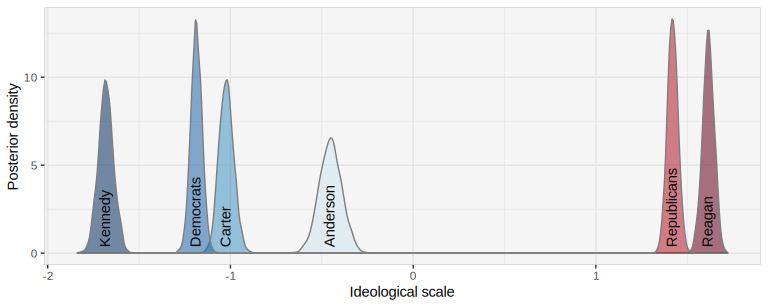In this example, we see that John B. Anderson – the former Republican who ran as an independent candidate – gets a wider posterior distribution, suggesting that voters were more uncertain about where to place him relative to the others.

The function plot_respondents() plots the distribution of estimated respondent positions. It illustrates the uncertainty of the estimates by calculating the population density for each of a set of posterior draws. The default is to use 15 draws for each respondent, but this can be altered by specifying the argument n_draws. The plot_respondents() function also plots the estimated stimulus positions by default, but this behavior can be turned off by adding the argument inc_stimuli = FALSE.

plot_respondents(fit_hbam, n_draws = 10)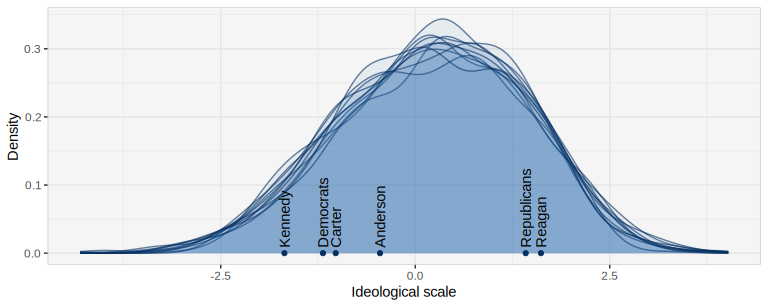Users who want to customize the plots further can obtain the underlying data by using the function get_plot_data(). This function accepts the same n_draws-argument as plot_respondents(). The output is a list of three tibbles: The first element contains the posterior mean stimulus positions, as well as the $$x$$- and $$y$$-values of the posterior modes (which can be useful for labeling the distributions). The second element contains the posterior draws for the stimulus positions (which can be used to calculate marginal posterior densities). The third element contains the selected number of posterior draws for each respondent (which form the key ingredient for the plot_respondents() function).

The function plot_over_self() plots the distributions of key parameter estimates over the respondents’ self-placements. In addition to a stanfit object produced by hbam(), this function requires the list of data produced by prep_data(). The function also takes the argument par, which can be either of the following: "alpha", "beta", "abs_beta", "lambda", "chi", and "eta". "abs_beta" calls for the absolute value of beta to be used. By default, the function uses posterior median estimates, but this can be changed by specifying estimate = "mean".

This function can, for instance, be used to assess the accuracy of respondents’ answers. In particular, when the argument par = "eta" is specified, the plotting function will display $$\sqrt{\eta_i} / J$$, which equals the average error for each individual (the mean of $$\sigma_{ij}$$ for each $$i$$ across $$j$$). The point estimates will still be calculated using the posterior median, unless the argument estimate = "mean" is added. (This plot is not available for the homoskedastic variants of the model (such as HBAM_MINI) as these do not estimate individual $$\eta$$ parameters.)

plot_over_self(fit_hbam, dat, "eta")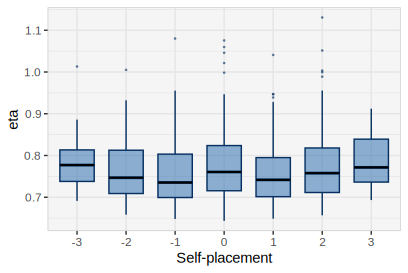The plot_over_self() function may also be used to compare results from several models by supplying the model fits as a list. To compare the distributions of estimated shift parameters from the HBAM and HBAM_MINI models, we would run:

plot_over_self(list(fit_hbam, fit_hbam_mini), dat, "alpha")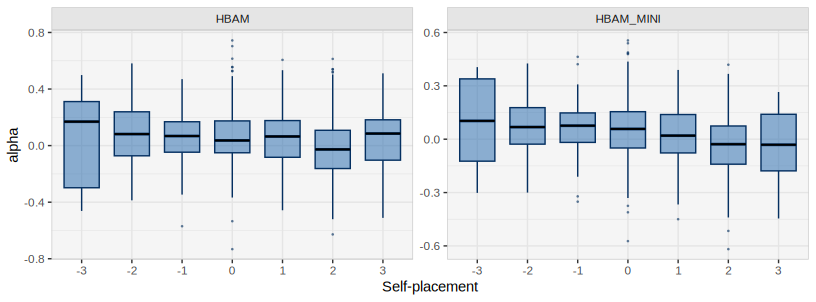For these models, the draws for $$\beta$$ combine the separate parameters for each flipping-state. The absolute value of $$\beta$$ may therefore be better suited for examining the extent to which each individual stretches the ideological space. To inspect the distribution of these values across self-placements, we would run:

plot_over_self(list(fit_hbam, fit_hbam_mini), dat, "abs_beta")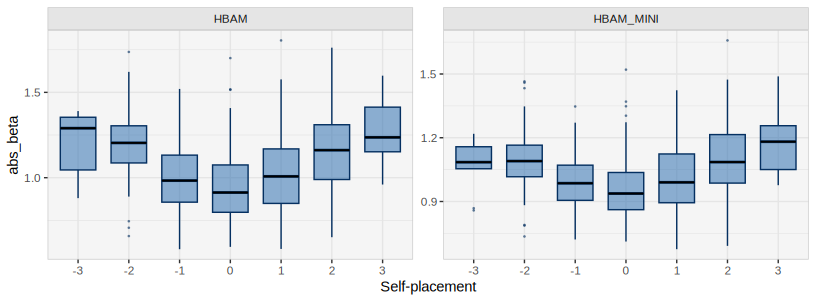The pattern above, where respondents with more extreme self-placements have more extreme $$\beta$$ parameters, is exactly the kind of differential item functioning that the models in this package are intended to correct for: These respondents tend to place both stimuli and themselves further out on the scale than others do, thus appearing more extreme in comparison.

To see whether these $$\beta$$ parameters are likely to be positive or negative, we can look at the expectation of the flipping parameters. These represent each respondent’s probability of not flipping the scale:

plot_over_self(list(fit_hbam, fit_hbam_mini), dat, "lambda")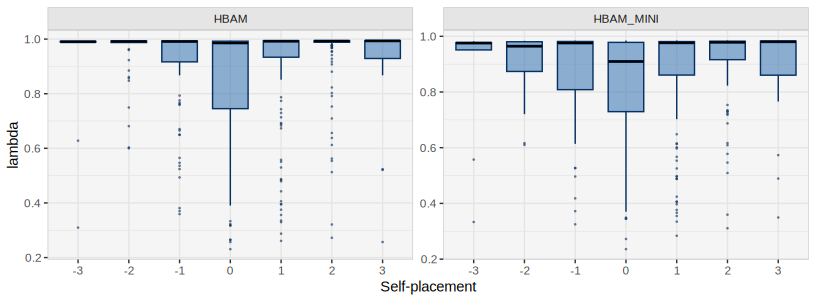In this example, flipping is very uncommon, but respondents who place themselves in the middle have a somewhat higher flipping-probability. This may suggest that some of these respondents are less informed about politics and provide less accurate answers.

It may also be useful to inspect the distribution of the scaled respondent positions over the self-placements. This illustrates the extent to which the model has transformed the original data. In this example, the impact of the models is generally modest, although a few respondents have been detected as having flipped the scale, and thus have had their self-placement flipped back.

plot_over_self(list(fit_hbam, fit_hbam_mini), dat, "chi")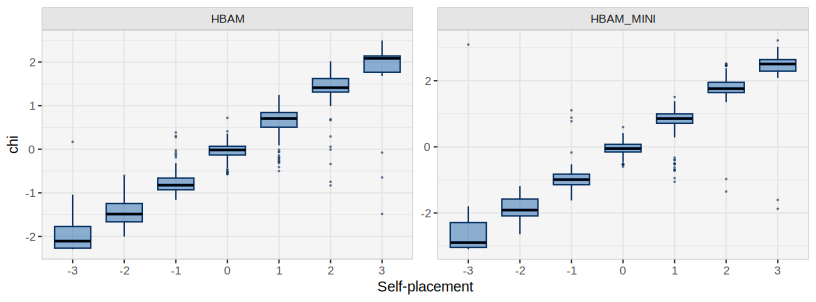## Posterior Summaries

The package also contains a wrapper for rstan::summary() called get_est(). This function takes the arguments object – a stanfit object produced by hbam() – and par – the name of the parameter(s) to be summarized. The function returns a tibble, which by default contains the posterior mean, the 95% credible interval, the posterior median, the estimated number of effective draws, and the split R-hat. One can obtain other posterior quantiles by using the argument probs. To get a 50% credible interval (and no median), one would add the argument probs = c(0.25, 0.75). To include the Monte Carlo standard error and the posterior standard deviation, use the argument simplify = FALSE.

The posterior draws for the stimulus positions can be summarized as follows:

get_est(fit_hbam, "theta")
## # A tibble: 6 × 6
##     mean 2.5%  50% 97.5% n_eff  Rhat
##    <dbl>  <dbl>  <dbl>   <dbl> <dbl> <dbl>
## 1 -1.02  -1.10  -1.02   -0.941 2160. 1.00
## 2  1.62   1.55   1.62    1.68  2013. 1.00
## 3 -1.68  -1.76  -1.68   -1.60  2473. 1.00
## 4 -0.453 -0.574 -0.453  -0.333 3159. 1.00
## 5  1.42   1.36   1.42    1.48  1912. 1.00
## 6 -1.19  -1.25  -1.19   -1.13  2404. 0.999

The equivalent call for the respondent positions is:

get_est(fit_hbam, "chi")
## # A tibble: 643 × 6
##       mean 2.5%    50% 97.5% n_eff  Rhat
##      <dbl>  <dbl>    <dbl>   <dbl> <dbl> <dbl>
##  1  0.965  -1.96   1.27      2.62  2862.  1.00
##  2  1.21   -0.613  1.26      2.57  3421.  1.00
##  3  1.39   -0.121  1.44      2.35  3212.  1.00
##  4  0.0128 -1.80   0.00469   1.76  3745.  1.00
##  5 -0.197  -2.04  -0.253     1.89  4089.  1.00
##  6  1.45   -1.78   1.68      2.94  2336.  1.00
##  7 -0.157  -1.15  -0.159     0.857 2975.  1.00
##  8  0.943  -0.738  0.991     2.01  2924.  1.00
##  9  0.587  -0.857  0.623     1.73  3691.  1.00
## 10  0.125  -1.02   0.136     1.14  3654.  1.00
## # ℹ 633 more rows

## Diagnostics

The rstan::sampling() function that hbam() uses automatically performs a number of key diagnostic checks after sampling and issues warnings when a potential issue is detected. The authors of rstan emphasize diagnostics and careful model development, and users of rstan will more frequently encounter warnings than users of rjags. One warning users of this package may encounter is that the Bulk or Tail Effective Sample Size (ESS) is too low (see https://mc-stan.org/misc/warnings.html). The most straightforward solution to this issue is to increase the number of posterior draws, using the iter argument. However, this increases the computational load, and users should consider carefully what level of accuracy they need.

Because the hbam() function returns a stanfit object, the model fit can be examined using the full range of diagnostic tools from the rstan package. Users should consult the rstan documentation for details on the various diagnostic tests and plots that are available. One example of the available tools is traceplot():

rstan::traceplot(fit_hbam, pars = "theta")